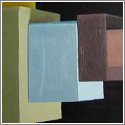• ## Validated ODE solvingSeveral space related problems can be formulated as ordinary differential equations. The aim of this project is to investigate self validated integration methods based on interval mathematics.

Traditional numerical techniques, as implemented on computers, use floating point calculations and thus introduce rounding errors which may lead to unacceptable inaccuracies and ultimately to mission failure. Applications such as space surveillance, Near Earth Objects hazard mitigation, collision avoidance manoeuvres, would benefit from approaches where predictions can mathematically be proven to be correct.

Self validated integration methods based  on interval mathematics seem to be promising techniques with this respect. The inclusion of the real solution of the problem considered is provided by these methods with mathematical rigor. However, the resulting inclusion could have overestimation, depending on the problem description and the method used to obtain the enclosure of the function. Reasonably tight inclusion of the solution needs some analysis (i.e. investigation of suitable formalism) and experiment on the problem and application of the proper techniques.

Many efficient enclosure methods and accelerating tools have been invented recently. There are general solvers available (VNODE , COSY , AWA , ValEncIA-IVP , VSPODE ) applying these methods to solve ODE problems in reliable way.

As first result of this project, a past Ariadna study  shows that usage of AWA and VNODE, as off-the-shelf solver, applied for space flight mechanical problems could lead pessimistic results in terms of large overestimation in the enclosure intervals of the solution.

Recent results show that validated ODE solvers based on Taylor models [3, 7], or based on calculation of state enclosures consisting of non-validated approximate solutions of the problem together with guaranteed error bounds for the approximation errors  are able to perform very well even on difficult problems ( and the references there, also [8,9]). These studies show that self validated solvers are able to reduce the wrapping effect and the dependency problem. The aim of this study is to investigate these new techniques and develop an efficient solver to have reasonable tight inclusion of the solution of selected space flight mechanics problems. To reach that point, a deep analysis on why the general interval solvers fail so early is needed.

## References

1. AWA
2. F. Bernelli Zazzera, M. Vasile, M. Massari, P. Di Lizia. “ Assessing the accuracy of interval arithmetic estimates in space flight mechanics”. Final report, Ariadna id: 04/4105, Contract Number: 18851/05/NL/MV, 2004.
3. M. Berz. “From Taylor series to Taylor models”. In AIP Conference Proceedings 405, pages 1–23, 1997.
4. COSY
5. G. F. Corliss. “Guaranteed error bounds for ordinary differential equations”. In Ainsworth, Levesley, Light and Marletta, editors, Theory of Numerics in Ordinary and Partial Differential Equations, pages 1-75. Oxford University Press, 1995.
6. Y. Lin and M. A. Stadtherr, “Validated Solutions of Initial Value Problems for Parametric ODEs,” to appear in Applied Numerical Mathematics, 2007.
7. K. Makino and M. Berz. “Taylor models and other validated functional inclusion methods”. Int. J. Pure Appl. Math., 4:379–456, 2003.
8. M. Neher. “Verified integration of linear n-th order ODEs using large steps”. Preprint 06/20, Fakultät für Mathematik, Universität Karlsruhe, 2006.
9. M. Neher, K. R. Jackson and N. S. Nedialkov. “On Taylor model based integration of ODEs”. Preprint 05/21, Fakultät für Mathematik, Universität Karlsruhe, 2005.
10. ValEncIA-IVP
11. VNODE

## Outcome

1. Zazzera, F.B., M. Vasile, M. Massari, and P. Di Lizia. 2004. “Assessing the Accuracy of Interval Arithmetic Estimates in Space Flight Mechanics.” 04-4105 European Space Agency, the Advanced Concepts Team. [link]